asum## asum

بازدید : 651 چهارشنبه 10 دي 1393

## ()asum

This function takes a sum over an X-Y region. The syntax is:

`asum(expr, xdim1, xdim2, ydim1, ydim2)`

where:

`expr    `- any valid grads expression
``` xdim1   - starting X dimension expression  ``` ``` xdim2   - ending X dimension expression  ``` ``` ydim1   - starting Y dimension expression  ``` ``` ydim2   - ending Y dimension expression  ```

For global summing, a shorthand may be used:

`asum(expr, global)` or
``` asum(expr, g) ```
is the same as
`asum(expr, lon=0, lon=360, lat=-90, lat=90)`

### Usage Notes

1. The `asum` function always does its average to the exact boundaries specified, in world coordinates. If the boundaries specified via the dimension expressions do not fall on grid box boundaries, then the values in the boundary grid boxes are weighted in the sum according to the length of the partial grid box in world coordinates.

2. If grid coordinates are used in the dimensions expressions, then they are converted to world coordinates to determine the exact boundary values. This conversion is done using the scaling of the default file. Note that the conversion is done using the outside grid box boundary, rather than the grid box center. For example:

`asum(expr,x=1,x=72,y=1,y=46)`

Here the boundary would be determined by using the X grid values ranging from 0.5 to 72.5 and Y grid values ranging from 0.5 to 46.5. These four grid boundary values would be converted to world coordinates using the scaling information from the default file. If we assume that `x=1` is 0 degrees longitude and `x=72` is 355 degrees longitude, then the averaging boundary would be -2.5 to 357.5 degrees, which would cover the earth. In the Y dimension, when the boundary is beyond the pole, the `asum` function recognizes this and weights appropriately. To calculate a sum without any weighting of partial grid boxes at the domain boundaries, use the `asumg` function.

3. There is no latitude-weighting of grid box values in `asum`. The `atot` function (in grads version 2.0.2+) will calculate a sum over an area and weight the grid box values according to the difference in the sine of the latitude at the northern and southern boundaries of each grid box.
مطالب مرتبط
ارسال نظر برای این مطلب

نام
ایمیل (منتشر نمی‌شود) (لازم)
وبسایت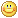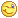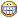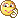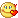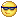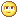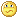نظر خصوصی
مشخصات شما ذخیره شود ؟ [حذف مشخصات] [شکلک ها]کد امنیتی
اطلاعات کاربری
 نام کاربری : رمز عبور :
• فراموشی رمز عبور؟
• لینک دوستان
آرشیو
آمار سایت
• کل مطالب : 155
• کل نظرات : 0
• افراد آنلاین : 1
• تعداد اعضا : 4
• آی پی امروز : 7
• آی پی دیروز : 17
• بازدید امروز : 75
• باردید دیروز : 81
• گوگل امروز : 0
• گوگل دیروز : 1
• بازدید هفته : 319
• بازدید ماه : 291
• بازدید سال : 36,465
• بازدید کلی : 157,517
• کدهای اختصاصی